BRONZE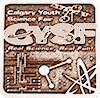#### A Ball's Flight Through Air

Study of the forces that develop when a spinning body travels through the air. Theory is presented as well as some expirements to demostrate the effect of the Magnus Force

### Hypothesis

Hypothesis: We feel that the ball will curve around and go to the the left/right depending which side of the ball launcher is covered

# A Balls’ Flight Through Air

## 1st Experiment: Experiment of Ball Flight; Ball Launcher

Question: Will the ball spin when it leaves the ball launcher?

Hypothesis:  We think that the ball will curve around and go to the left/right depending which side is covered.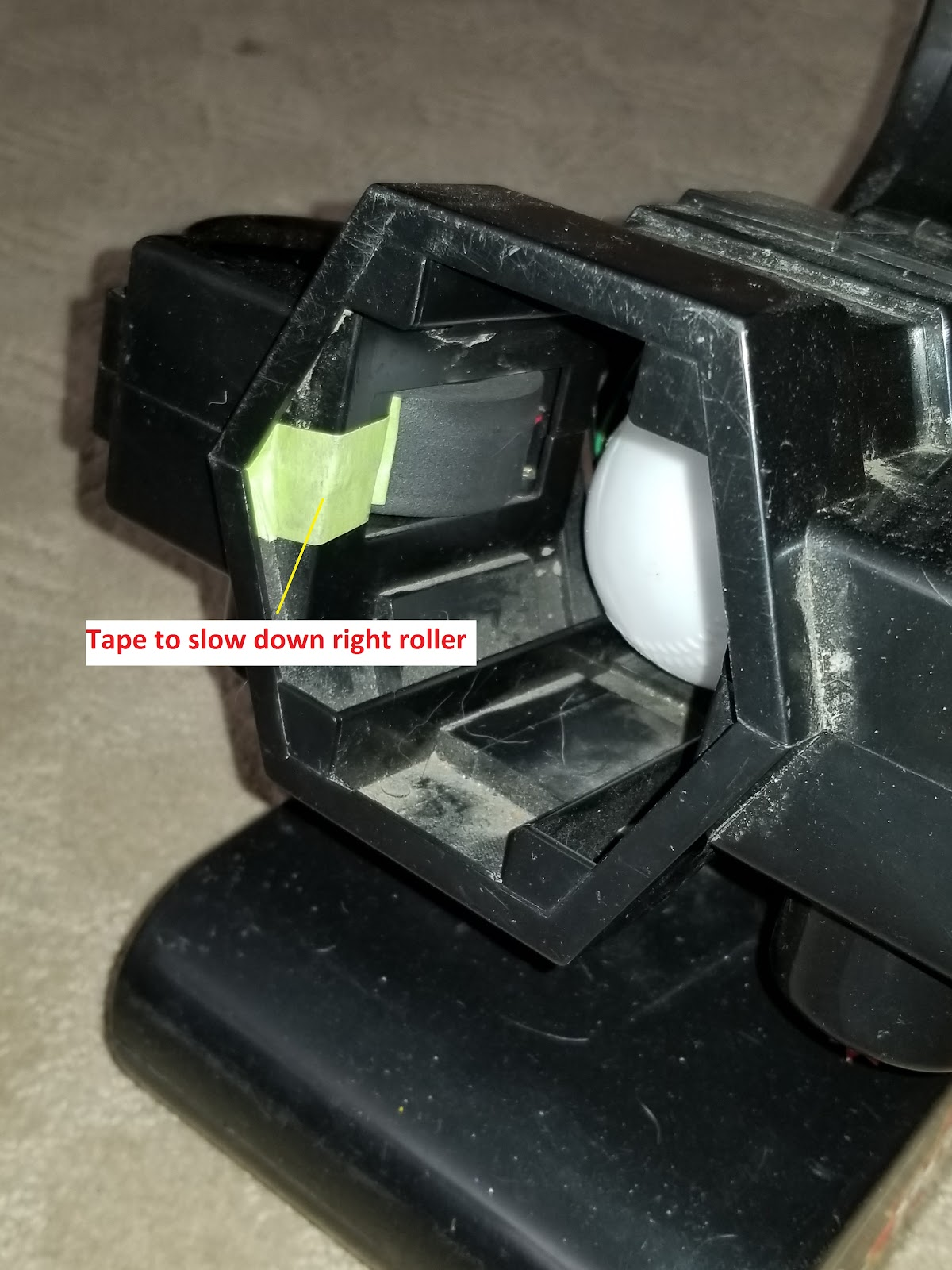Here is the video of our 1st experiment

Conclusion:

Yes our hypothesis was correct because when the ball was launched from the ball launcher it curved to the right because the right side was covered

### What is the Magnus Effect?

When a ball is moving forwards while spinning through the air. Air is flowing from the front to the back of the ball (The ball is spinning such that the top moves in the same direction as the flow and the bottom moves opposite to the flow).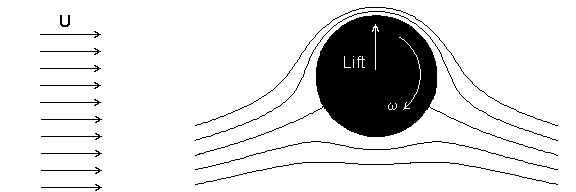Due to friction between the air and the ball’s surface, air is dragged around the top of the ball, downwards towards the back of the ball.  At the bottom of the ball, the air flows and the ball moves in opposite directions. Air at the bottom of the ball comes to a halt instead of being deflected upwards. The result is that air is deflected downwards, and due to Newton’s third law (Every force has an opposite and equal reaction) the air exerts an equal opposite force upwards on the ball (Lift), similarly to what happens to a wing when it moves through the air. This force makes the ball go farther. If the ball spins on its vertical axis, the force will pull the ball to one side like in the experiment.

### How the Ball Launcher creates lift

When a ball is spinning through the air it is affected by a force called Magnus Force. When the ball was spinning it’s path was  different from when the ball was not spinning. As a ball spins when it goes through the air, because of the Magnus Effect the ball is pulled to one side, describing a curved trajectory instead of going straight in the direction in which the ball was pointed. Inside the Ball Launcher there are two rollers that make the ball go forwards (thrust) but if one roller is slowed down then the ball rotates as it goes forward. The rotation creates lift and deflects the ball off it’s path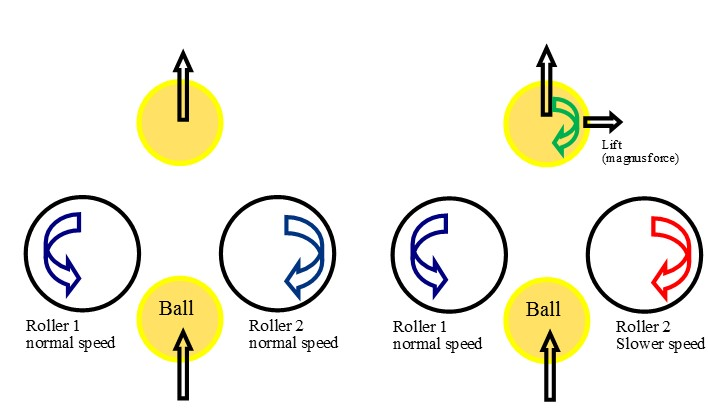#### Magnus Effect: A Second Experiment

We did a second experiment because we wanted to see what would happen if we change one of the variables.We changed our variable from a baseball to a paper roll. Since a paper roll is lighter, we wanted to see the difference in the curve.

Video of our 2nd experiment

Results of the 2nd experiment:

When a Tennis Ball rolls off an inclined surface, you expect it to go in a parabolic arc but if we take a light, cylindrical object, like a roll of paper the results are much different as shown in the picture below. This is a video of our second experiment.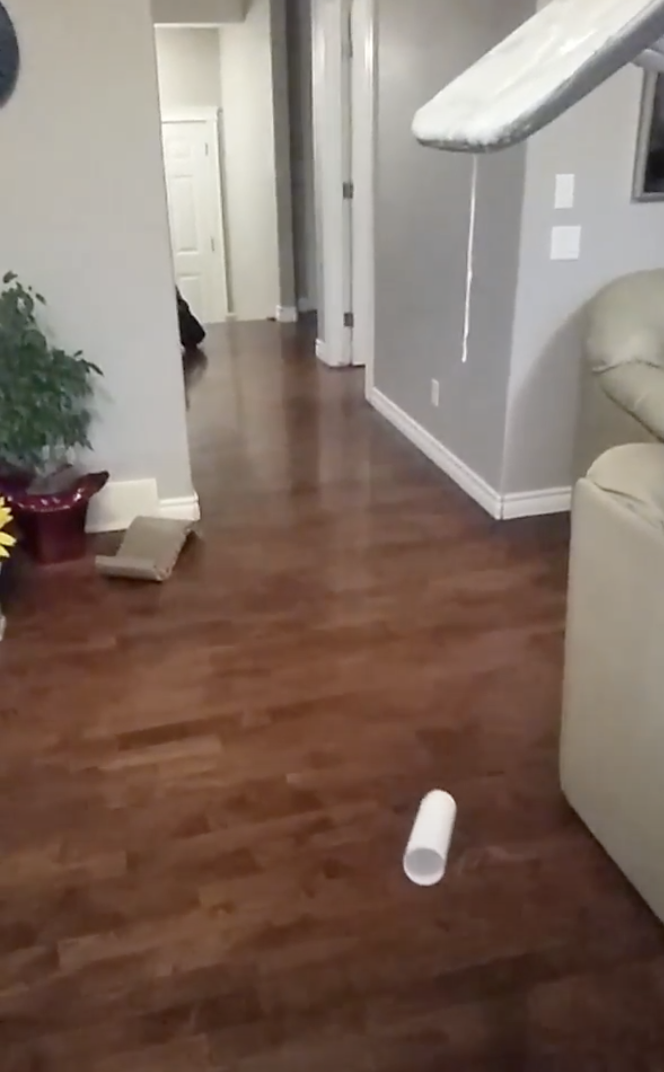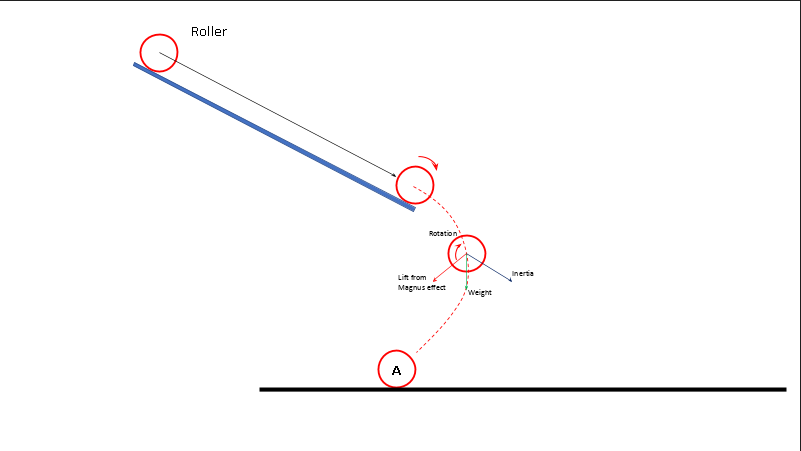From this diagram we can observe all the contributing factors of why the paper roll goes the way it does.

### Joukowski theorem:

The Joukowski theorem can be used to calculate the lift (The Upwards Force) of a rotating cylinder, with a simple formula, As explained in this image

The Joukowski Theorem is a mathematical model (G = 2ρωr2) of what happens in reality with the Magnus Effect .

In the early years of aviation it was used to design the shape of airfoils.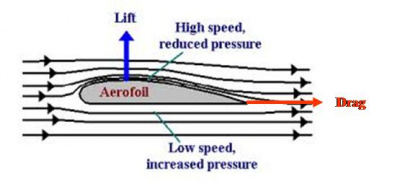### Variables

We could have made one paper roll that doesn't spin and just drop it straight in the air while the other one spins, and I think it would have made the project a bit better.

### Procedure

We Had a ball launcher and covered one side of it to make it spin, then we collected the data and researched it. We also did an expirement in which we rolled a roll of paper down an  inclined surface, then repeated the process ( collected data then researched)

### Observations

When the roll of paper slowly rolled down the ironing board we noticed that it went inwards, towards the opposite direction in which it was rolled.

### Analysis

It disagreed with our Hypothesis because we I thought it would roll forwars the direction it was being rolled. The Magnus Effect does this because when a ball is moving forwards while spinning through the air. Air is flowing from the front to the back of the ball (The ball is spinning such that the top moves in the same direction as the flow and the bottom move opposite to the flow) due to friction between the air and the ball’s surface, air is dragged around the top of the ball, downwards towards the back of the ball.  At the bottom of the ball, the air flows and the ball moves in opposite directions. Air at the bottom of the ball comes to a halt fairly soon, instead of being deflected upwards. The result is that air is deflected downwards, and due to Newton’s third law (Every force has an opposite reaction) the air exerts an equal opposite force upwards on the ball (Lift), similarly to what happens to a wing when it moves through the air. This force makes the ball go farther. If the ball spins on its vertical axis, the force will pull the ball to one side like in the experiment.

One research question is that does the Magnus Effect work underwater? and we could have expanded our project by maybe using the Joukowski theorum to calculate path of movement, from a paper roll.

### Conclusion

We learned that the Magnus Effect makes a object Move in the air unpredictably, and not the direction was headed, sort of like a curveball in football/soccer.

### Application

Use in real world

Curveball

Skydiving

Bowling pins

Recycling things

Planes

### Sources Of Error

I don't think we went wrong anywhere but I think we could have expaned our project more by adding more experiments and the what-if's to it.

### Acknowledgement

Thank Diego and parents and stuff thanks NASA for pic of airfoil and thank HG magnus for figuring this out